# Fourier transform of differentials equation

naspek
hey there.. i really dont know how to start answering this question.. can someone please guide me to solve this question..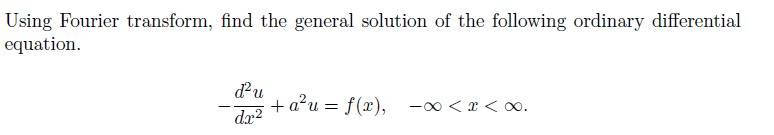## Answers and Replies

Homework Helper
Gold Member
Since the question tells you to use Fourier transforms, you start by taking the FT of both sides of the differential equation...

naspek
Since the question tells you to use Fourier transforms, you start by taking the FT of both sides of the differential equation...
still blur...Homework Helper
Gold Member
$$F\left[-\frac{d^2u}{dx^2}+a^2u\right]=F\left[-\frac{d^2u}{dx^2}\right]+F\left[a^2u\right]=F[f(x)][/itex] Call the FT of $f(x)$, $F[f(x)]=\tilde{f}(k)$. Call the FT of $u(x)$, $F[u(x)]=\tilde{u}(k)$. Your textbook likely tells you how to express the Fourier transform of $-\frac{d^2u}{dx^2}$ and $a^2u(x)$ in terms of $\tilde{u}(k)$....do exactly that naspek i dont know how to proceed from here... [tex]F\left[-\frac{d^2u}{dx^2}+a^2u\right]=F\left[-\frac{d^2u}{dx^2}\right]+F\left[a^2u\right]=F[f(x)]$$

$$F\left[-\frac{d^2u}{dx^2}+a^2u\right]=F\left[-\frac{d^2u}{dx^2}\right]+a^2F\left[u\right]=F[f(x)]$$

$$F\left[-\frac{d^2u}{dx^2}+a^2u\right]=F\left[-(-k^2\tilde{u}(k,y))\right]+a^2F\left[u\right]=F[f(x)]$$

Homework Helper
Gold Member
When you take the fourier transform of $-\frac{d^2u}{dx^2}$ (That's what the $F[\ldots]$ means) you get $k^2F$, or $k^2\tilde{u}(k)$.

This gives you an algebraic (instead of differential) equation you can solve for $\tilde{u}$. To find $u$, you then take the inverse FT.

This is a very basic application of using FTs to solve DEs and I have a hard time believing that your textbook doesn't provide a similar example. You should really be in the habit of studying the relevant section of your text/notes before attempting homework problems that are based off of it.

naspek
i got the general solution already.. howeverr.. how am i going to prove...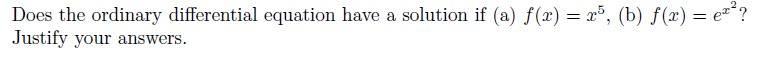naspek
When you take the fourier transform of $-\frac{d^2u}{dx^2}$ (That's what the $F[\ldots]$ means) you get $k^2F$, or $k^2\tilde{u}(k)$.

This gives you an algebraic (instead of differential) equation you can solve for $\tilde{u}$. To find $u$, you then take the inverse FT.

This is a very basic application of using FTs to solve DEs and I have a hard time believing that your textbook doesn't provide a similar example. You should really be in the habit of studying the relevant section of your text/notes before attempting homework problems that are based off of it.

i've done the inverse..

F^-1[g^(k)] = g(x) = 1/ √2∏ ∫g^(k)e^ikx dk
.............................. = 1/ √2∏ ∫ 1/ (k^2 + a^2)e^ikx dk
...............................= √2∏ [(e^-a|x|) / 2a]

Homework Helper
Gold Member
i've done the inverse..

F^-1[g^(k)] = g(x) = 1/ √2∏ ∫g^(k)e^ikx dk
.............................. = 1/ √2∏ ∫ 1/ (k^2 + a^2)e^ikx dk
...............................= √2∏ [(e^-a|x|) / 2a]

$$\tilde{u}(k)\neq\frac{1}{k^2+a^2}$$

What happened to the FT of $f(x)$?

naspek
accidently double post

Last edited:
naspek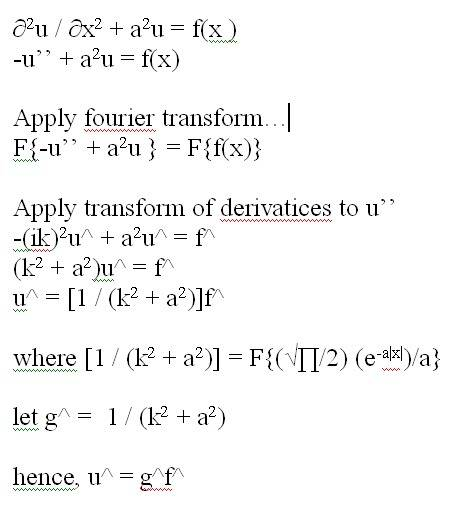ok.. this is my full solution...
am i got it right until here?

Homework Helper
Gold Member
That looks good, now use the composition rule to take the inverse FT of [tex]\hat{u}=\hat{g}\hat{f}[/itex]...

naspek
That looks good, now use the composition rule to take the inverse FT of [tex]\hat{u}=\hat{g}\hat{f}[/itex]...

inverse both side...
i get..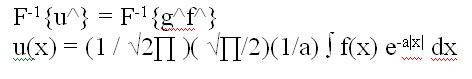is that my general solution?

Homework Helper
Gold Member
Does $\frac{1}{2a}\int f(x) e^{-a|x|}dx$ really represent the convolution of $f$ and $g$?

naspek
That looks good, now use the composition rule to take the inverse FT of [tex]\hat{u}=\hat{g}\hat{f}[/itex]...

Does $\frac{1}{2a}\int f(x) e^{-a|x|}dx$ really represent the convolution of $f$ and $g$?

by convolution theorem,
F^−1{F*G} = f ·g
correct?
so, i just substitute it..?
kinda confusednaspek
ok.. i revised bout it already..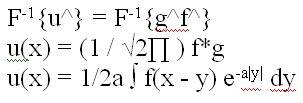Homework Helper
Gold Member
That's better!However, that's just part of the general solution. You can also add any function, $u_h$, which satisfies the corresponding homogeneous ODE, $-\frac{d^2 u_h}{dx^2}+a^2u_h=0$, to the $u(x)$ you've found, and the result will still satisfy your ODE. So, you need to find that homogeneous solution and add it to what you've found above in order to obtain the true general solution.

naspek
That's better!However, that's just part of the general solution. You can also add any function, $u_h$, which satisfies the corresponding homogeneous ODE, $-\frac{d^2 u_h}{dx^2}+a^2u_h=0$, to the $u(x)$ you've found, and the result will still satisfy your ODE. So, you need to find that homogeneous solution and add it to what you've found above in order to obtain the true general solution.

should i solve the integration u(x) = 1/2a ∫ f(x - y) e^-a|y| dy?

Homework Helper
Gold Member
First worry about finding the homogeneous part of the general solution. Solve the Homogeneous equation.

naspek
First worry about finding the homogeneous part of the general solution. Solve the Homogeneous equation.

can u give me more clue.. i really can't get it..
which homogeneous equation i need to solve?

Homework Helper
Gold Member

naspek
is this my general solution?

$y(x)=\frac{1}{\sqrt{2\pi}}\frac{1}{a}\sqrt{\frac{\pi}{a}}\int_{-\infty}^{\infty} e^{-a|x|}f(x-t)dt$

naspek
now.. to answer my question, u'' + a^2u = x^5
after solving everything, i manage to get this equation..

$$\displaystyle u(x)= ∫- ∞ to 0 1/2a(x-y)^{5}e^{ay}dy+ ∫0 to ∞ (x-y)^{5}e^{-ay}dy should i solve it to get the general solution? because, i need to do integration by parts 5 times to solve it..$$

Homework Helper
Gold Member
is this my general solution?

$y(x)=\frac{1}{\sqrt{2\pi}}\frac{1}{a}\sqrt{\frac{\pi}{a}}\int_{-\infty}^{\infty} e^{-a|x|}f(x-t)dt$

$u(x)=\frac{1}{2a}\int_{-\infty}^{\infty} e^{-a|x|}f(x-t)dt$ is part of your general solution. You are missing the homogeneous part.

Once you find the true general solution, you need only determine whether the integrals involved in exist/converge for your two $f(x)$'s to know whether or not a solution exists.

naspek
this is my general solution?

$u(x)=c_1 e^{-ax}+c_2 e^{ax}+y(x)$

Homework Helper
Gold Member
Yup!naspek
Yup!fuuhh!! (just for a moment..)
so.. now i know how is my general equation looks like..

still got problem here..
i'm having difficulties to solve y(x) when i substitute f(x) = x^5

y(x)= ∫- ∞ to 0
1/2a(x-y)^{5}e^{ay}dy+
∫0 to ∞
(x-y)^{5}e^{-ay}dy

should i solved it?

Homework Helper
Gold Member
Are you asked to solve it? Or are you only asked to show that a solution exists? If it's the latter option, just show that the integral converges.

naspek
Are you asked to solve it? Or are you only asked to show that a solution exists? If it's the latter option, just show that the integral converges.

i try to solve it.. but.. its kinda to long for the solution..

the question ask me to find the general soltuion first..
then find when f(x) = x^5 & e^(x^2) exist or not,
if yes, i need to justify my answer..

how am i suppose to do right now? i kinda lost here..
right now, my general solution is...
$u(x)=c_1 e^{-ax}+c_2 e^{ax}+\frac{1}{\sqrt{2\pi}}\frac{1}{a}\sqrt{\frac{\ pi}{a}}\int_{-\infty}^{\infty} e^{-a|x|}f(x-t)dt$

how am i going to check whether the u(x) f(x) = x^5 will converges or diverges?

Homework Helper
Gold Member
Haven't you studied any convergence tests for improper integrals?

naspek
should i test for e^-ax or x^5 or e^(x^2) or
$y(x)=\int_{-\infty}^{\infty} e^{-a|x|}f(x-t)dt$
i'm sorry coz keep on asking.. but, i really dont know which one should i use to run the test.

Homework Helper
Gold Member
You want to test to see if $y(x)$ converges for each of your two $f(x)$'s. If the integral doesn't converge, then no solution exists.

naspek
You want to test to see if $y(x)$ converges for each of your two $f(x)$'s. If the integral doesn't converge, then no solution exists.
so.. i need to test the equations below?
$y(x)=\int_{-\infty}^{\infty} e^{-a|x|}f(x-t)^{5}dt$

and

$y(x)=\int_{-\infty}^{\infty} e^{-a|x|}f(e^{(x-t)^{2}})dt$

correct?

naspek
You want to test to see if $y(x)$ converges for each of your two $f(x)$'s. If the integral doesn't converge, then no solution exists.

by performing the improper integral test, when f(x) = x^5, the integral will converges
when f(x) = e^(x^2), the integral will diverges..

hence.. when f(x) = x^5, the is a solution, when f(x) = e^(x^2), there is no solution..
correct?

Homework Helper
Gold Member
I just noticed that you have an error in your expression. Convolution tells you that $y(x)=\frac{1}{2a}\int_{-\infty}^{\infty}f(x-t)e^{-a|t|}dt$, not $\frac{1}{2a}\int_{-\infty}^{\infty}f(x-t)e^{-a|x|}dt$.